## Median (Triangle)The Cevian from a Triangle's Vertex to the Midpoint of the opposite side is called a median of the Triangle. The three medians of any Triangle are Concurrent, meeting in the Triangle's Centroid (which has Trilinear Coordinates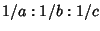). In addition, the medians of a Triangle divide one another in the ratio 2:1. A median also bisects the Area of a Triangle.

Letdenote the length of the median of theth side. Then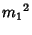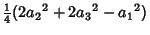(1)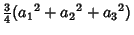(2)

(Johnson 1929, p. 68). The Area of a Triangle can be expressed in terms of the medians by(3)

where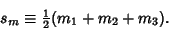(4)

A median triangle is a Triangle whose sides are equal and Parallel to the medians of a given Triangle. The median triangle of the median triangle is similar to the given Triangle in the ratio 3/4.

References

Casey, J. A Sequel to the First Six Books of the Elements of Euclid, Containing an Easy Introduction to Modern Geometry with Numerous Examples, 5th ed., rev. enl. Dublin: Hodges, Figgis, & Co., 1888.

Coxeter, H. S. M. and Greitzer, S. L. Geometry Revisited. Washington, DC: Math. Assoc. Amer., pp. 7-8, 1967.

Johnson, R. A. Modern Geometry: An Elementary Treatise on the Geometry of the Triangle and the Circle. Boston, MA: Houghton Mifflin, pp. 68, 173-175, 282-283, 1929.